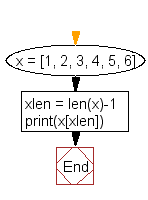﻿ Python: Check whether the n-th element exists in a given list - w3resource# Python: Check whether the n-th element exists in a given list

## Python List: Exercise - 59 with Solution

Write a Python program to check whether the n-th element exists in a given list.

Sample Solution:-

Python Code:

``````x = [1, 2, 3, 4, 5, 6]
xlen = len(x)-1
print(x[xlen])
```
```

Sample Output:

```6
```

Pictorial Presentation:Flowchart:## Visualize Python code execution:

The following tool visualize what the computer is doing step-by-step as it executes the said program:

Python Code Editor:

Have another way to solve this solution? Contribute your code (and comments) through Disqus.

What is the difficulty level of this exercise?

Test your Python skills with w3resource's quiz

﻿

## Python: Tips of the Day

Floor Division:

When we speak of division we normally mean (/) float division operator, this will give a precise result in float format with decimals.

For a rounded integer result there is (//) floor division operator in Python. Floor division will only give integer results that are round numbers.

```print(1000 // 300)
print(1000 / 300)```

Output:

```3
3.3333333333333335```